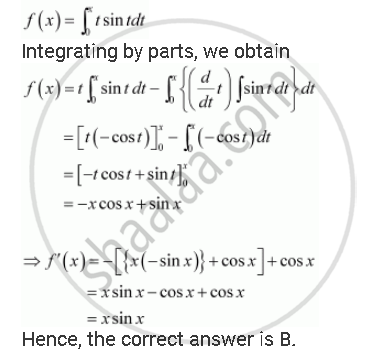Share
Notifications

View all notifications
Books Shortlist
Your shortlist is empty

# Solution for If F(X) = Int_0^Pi T Sin T Dt, Then F (X) is - CBSE (Science) Class 12 - Mathematics

Login
Create free account

Forgot password?
ConceptEvaluation of Definite Integrals by Substitution

#### Question

if f(x) = int_0^pi t sin t dt, then f' (x) is

A. cos x + x sin x

B. x sin x

C. x cos x

D. sin x cos x

#### SolutionIs there an error in this question or solution?

#### Video TutorialsVIEW ALL 

Solution If F(X) = Int_0^Pi T Sin T Dt, Then F (X) is Concept: Evaluation of Definite Integrals by Substitution.
S